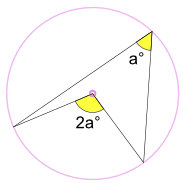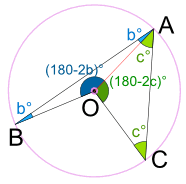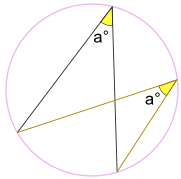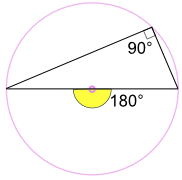# Theorems, Corollaries, Lemmas

What are all those things? They sound so impressive!

Well, they are basically just facts: some result that has been arrived at.

• A Theorem is a major result
• A Corollary is a theorem that follows on from another theorem
• A Lemma is a small result (less important than a theorem)

## Examples

Here is an example from Geometry:

### Example: A Theorem and a Corollary

#### Theorem:#### Corollary:

Following on from that theorem we find that where two lines intersect, the angles opposite each other (called Vertical Angles) are equal (a=c and b=d in the diagram).Angle a = angle c
Angle b = angle d

#### Proof:

Angles a and b add to 180° because they are along a line:

a + b = 180°

a = 180° − b

Likewise for angles b and c

b + c = 180°

c = 180° − b

And since both a and c equal 180° − b, then

a = c

And a slightly more complicated example from Geometry:

### Example: A Theorem, a Corollary to it, and also a Lemma!

#### Theorem:An inscribed angle a° is half of the central angle 2a°

Called the Angle at the Center Theorem.

Proof: Join the center O to A.Triangle ABO is isosceles (two equal sides, two equal angles), so:

Angle OBA = Angle BAO =

And, using Angles of a Triangle add to 180°:

Angle AOB = (180 − 2b)°

Triangle ACO is isosceles, so:

Angle OCA = Angle CAO =

And, using Angles of a Triangle add to 180°:

Angle AOC = (180 − 2c)°

And, using Angles around a point add to 360°:

Angle BOC = 360° − (180 − 2b)° − (180 − 2c)°
= 2b° + 2c°
= 2(b + c)°

Replace b + c with a, we get:

Angle BAC = a° and Angle BOC = 2a°And we have proved the theorem.

(That was a "major" result, so is a Theorem.)

#### Corollary

(This is called the "Angles Subtended by the Same Arc Theorem", but it’s really just a Corollary of the "Angle at the Center Theorem")

Keeping the endpoints fixed ... ... the angle a° is always the same, no matter where it is on the circumference:So, Angles Subtended by the Same Arc are equal.

#### Lemma

(This is sometimes called the "Angle in the Semicircle Theorem", but it’s really just a Lemma to the "Angle at the Center Theorem")In the special case where the central angle forms a diameter of the circle:

2a° = 180° , so a° = 90°

So an angle inscribed in a semicircle is always a right angle.

(That was a "small" result, so it is a Lemma.)

Another example, related to Pythagoras' Theorem:

### Example:

#### Theorem

If m and n are any two whole numbers and

• a = m2 − n2
• b = 2mn
• c = m2 + n2

then a2 + b2 = c2

Proof:

a2 + b2 = (m2 − n2)2 + (2mn)2
= m4 − 2m2n2 + n4 + 4m2n2
= m4 + 2m2n2 + n4
= (m2 + n2)2
= c2

(That was a "major" result.)

#### Corollary

a, b and c, as defined above, are a Pythagorean Triple

Proof:

From the Theorem a2 + b2 = c2,
so a, b and c are a Pythagorean Triple

(That result "followed on" from the previous Theorem.)

#### Lemma

If m = 2 and n = 1, then we get the Pythagorean triple 3, 4 and 5

Proof:

If m = 2 and n = 1, then

• a = 22 − 12 = 4 − 1 = 3
• b = 2 × 2 × 1 = 4
• c = 22 + 12 = 4 + 1 = 5

(That was a "small" result.)